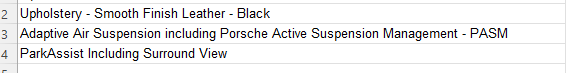# Writing DataTable rows as items in array

Hey there,

I have a Data Table of values which I want to write into an array but can’t seem to get it working. Essentially, row(0) has different values I want in an array of strings.

I have started with a ‘for each row’ for the DT but I can’t quite figure out how I get these together as an array of strings?

Hi @dr1992 ,

Could you let us know what is the format of output that you require ?

Based on this statement, maybe we can assume you have multiple data in a single cell. We would need to identify what is the separator for each of the values present, So that we can perform a Split operation based on that separator.

However, to simply get all the column values present in the row. We can use the below :

``````row.ItemArray.Select(Function(x)x.ToString).ToArray
``````

Above expression, gets the values present in the row as a Array of String .

1 Like

Hey,

Thanks for your reply; so I trued the suggested and it gave the three column items as an array, however, what I require is to have each row of the first column as an item within the array.

Eg, the data table:

I require the 3 highlighted options (upholstery, adaptive air suspension & ParkAssist as 3 items in one array, whereas the suggested game me the upholstery, retail price and monthly price.

Although there is a brief understanding of the logic that is to be performed to achieve this output. We do not the complete explanation as to why you want only the Highlighted values and not `-PASM` or `Optional Extras` ?

Could you also maybe provide an Input Excel Data and it’s Expected Output Data for us to confirm on the logic that would need to be applied ?

It scrapes like this:

But I just want the following in an array:Give a try on the below Expression :

``````strArray = DT.AsEnumerable.Select(Function(x)x(0).ToString).ToArray
``````

Where `strArray` is of the type Array of String, `DT` is your Input Datatable variable.

Let us know if the result is not as expected.

1 Like

Blockquote

That is exactly what I needed. Very much appreciate!

1 Like

This topic was automatically closed 3 days after the last reply. New replies are no longer allowed.